Open-Domain Compositional Image Editing with Text
 Zhizhuo Zhou Gaoyue Zhou Qiyun Chen
 [Demo] [Slides]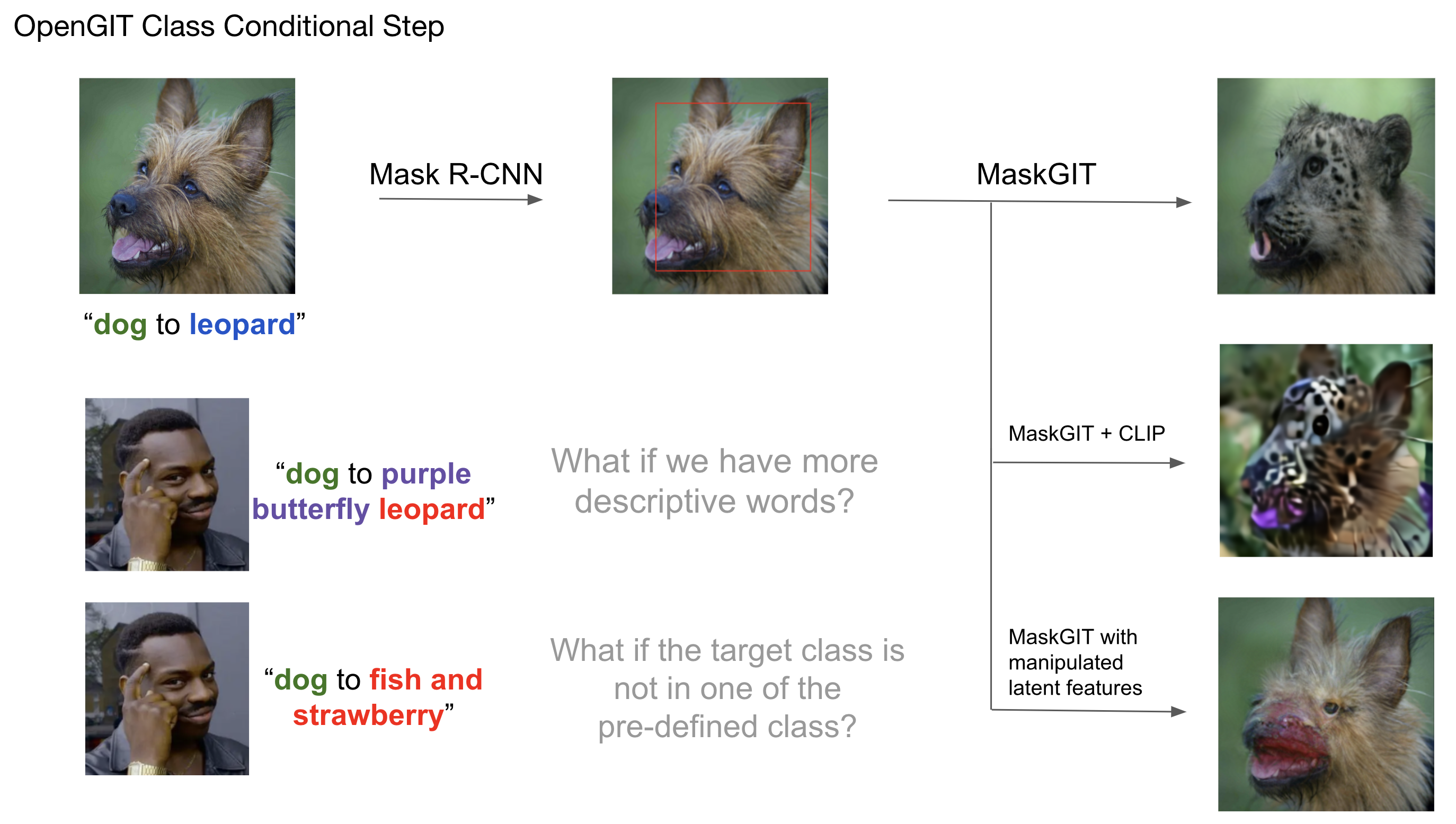This is a final course project for 16-726 Learning Based Image Synthesis in Spring 2022.

# Abstract

 Building on top of a recent class conditional generative image transformer, MaskGIT, we propose to develop a pipeline for language controlled image editing. We focus on a simple yet ubiquitous logic, {a} to {b}, which can be expressed as "change {a} to {b}" or "replace {a} with {b}", and apply the desired actions by performing edits on an input image. We constrain {a} to be a class in COCO and {b} to be an open-domain phrase that describes an object. Finally, we leverage Mask R-CNN, MaskGIT, and CLIP to perform region targeted class conditional image generation and image-language optimization.

# Method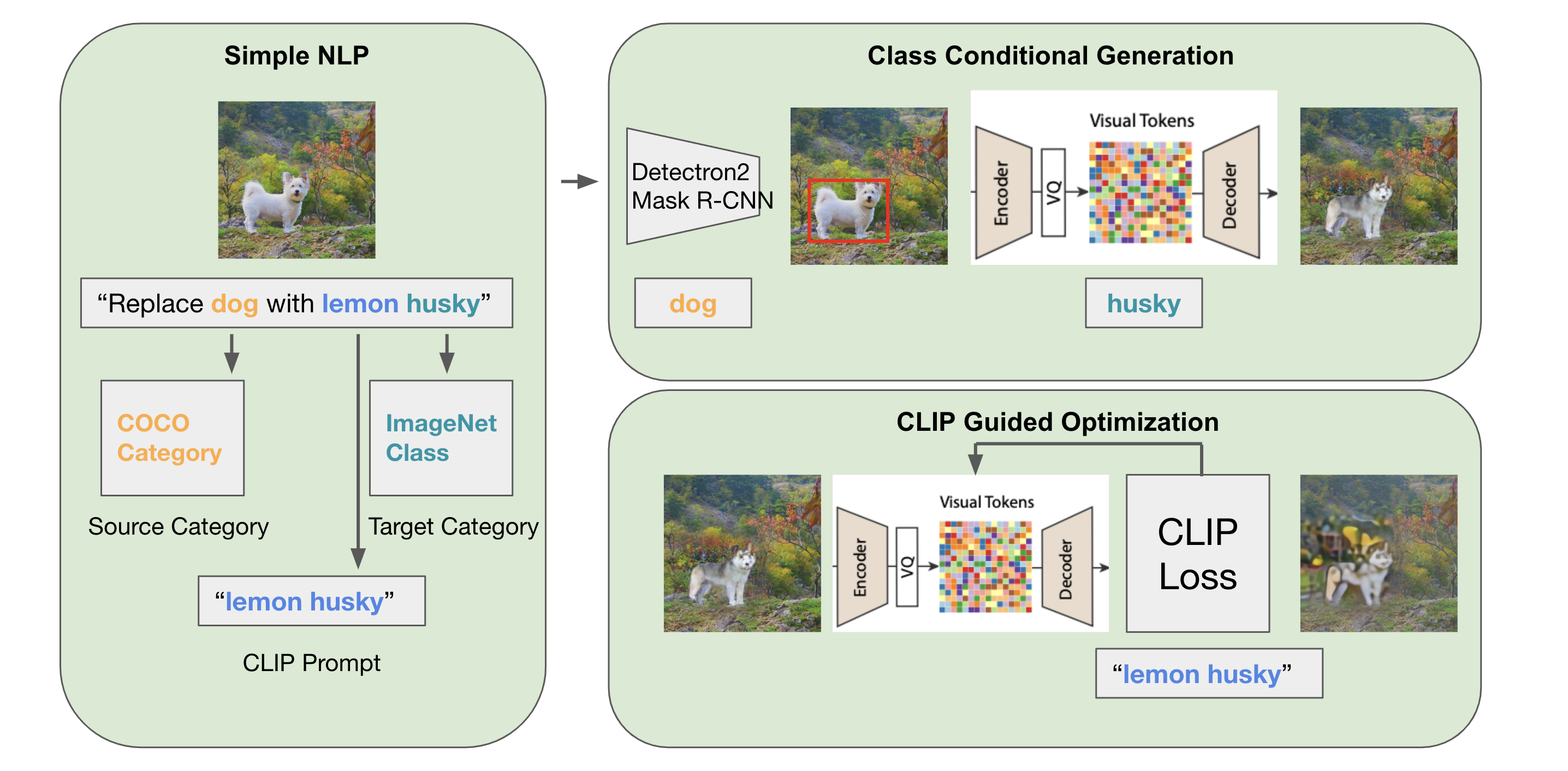OpenGIT Pipeline
 Our proposed OpenGIT Pipeline consists of three main components: Natural Language Processing Module, Class Conditional Image Generation, and CLIP Guided Optimization. The entire procedure is as follows: Firstly, OpenGIT performs Natural Language Processing (NLP), the user inputs an image and a natural language prompt specifying the source category (the item to be replaced in the input image) and the target category ( the item to change into). The source category must be from one of the categories in the COCODataset . The main target category (or categories) should be within the 1000 lables in the ImageNet Dataset. However, it can be a simple category like "husky", or it can also be any open-domain phrase that describes the category (categories), such as "zebra-striped cat" or "fish and strawberry". As an illustration for the first module, the user could input an image of a dog and a prompt "Replace dog with lemon husky". Then, our module would parse "dog" as our source category, "husky" as our target category, and "lemon husky" as our CLIP prompt. Here, note that because we used a descriptive word for our target category, we would use CLIP to further optimize our image. Otherwise, if the input prompt merely said "Replace dog with husky", then we would not have any CLIP prompt nor perform CLIP optimization. Secondly, OpenGIT performs Class Conditional Generation. This module take as inputs the input image along with the source and target category. The module first uses Detectron2 and Mask R-CNN to locate the bounding box of the source category, and then feed it through a pretrained MaskGIT model to generate a class conditioned image. Here, we offer users to choose a hyperparameter $r \in (0, 1]$ as the scale of the original bounding box. For example, in general, if $r = 1$, then OpenGIT would completely replace the source category with the target category. If $r < 1$, then OpenGIT would keep the outerior of the source category and merges the target category into the source category (see examples in Results section below). Thirdly, OpenGIT performs CLIP Guided Optimization. For the user input prompts with a descriptive prompt for the target category, the first NLP module would obtain a non-empty CLIP prompt. We take our class conditioned generated output and the CLIP prompt as the input to this module, and we use a pretrained CLIP module to generate the output image.

# Modules

### Object Detection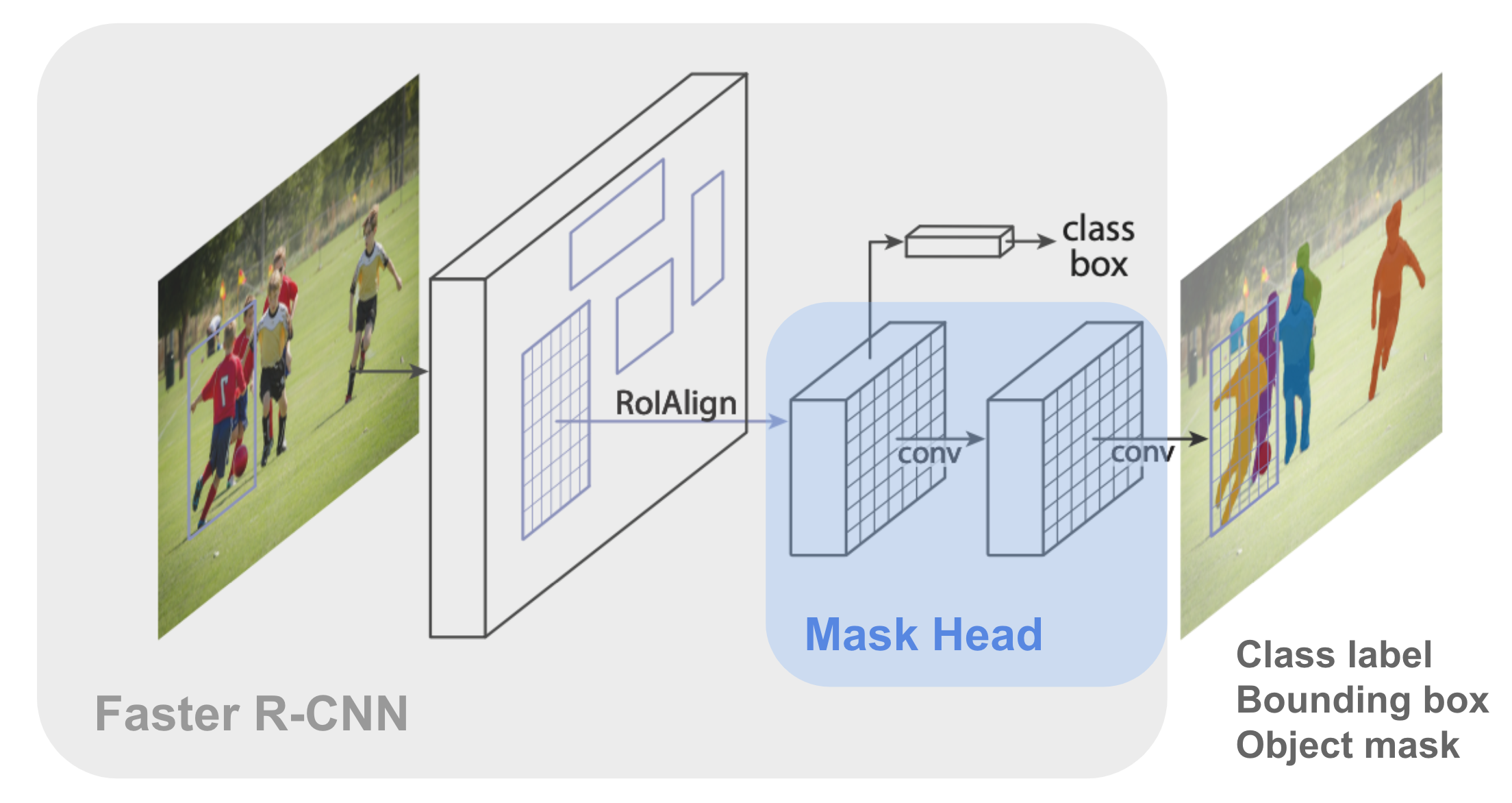Once we get the object class to be replaced by parsing the user prompt, we use a MaskRCNN model pretained on the COCO dataset to predict the bounding box of the specified object in the input image. The above figure shows the model structure of MaskRCNN. MaskRCNN is an extension of Faster R-CNN with an additional branch for predicting segmentation masks on each region of interest. The model has two stages. During the first stage, regions of interest are proposed by the region proposal network. These will be further processed by the Region of Interest Pooling networks to exact features and perform object classification. In parallel to object classification, binary masks of objects are predicted by the Mask Head portion denoted in the figure. The outputs of MaskRCNN include class labels, bounding boxes, and object masks for all objects. In OpenGIT, we make use of the bounding box of the specified object for later processing.

### Class-Conditioned Image Editing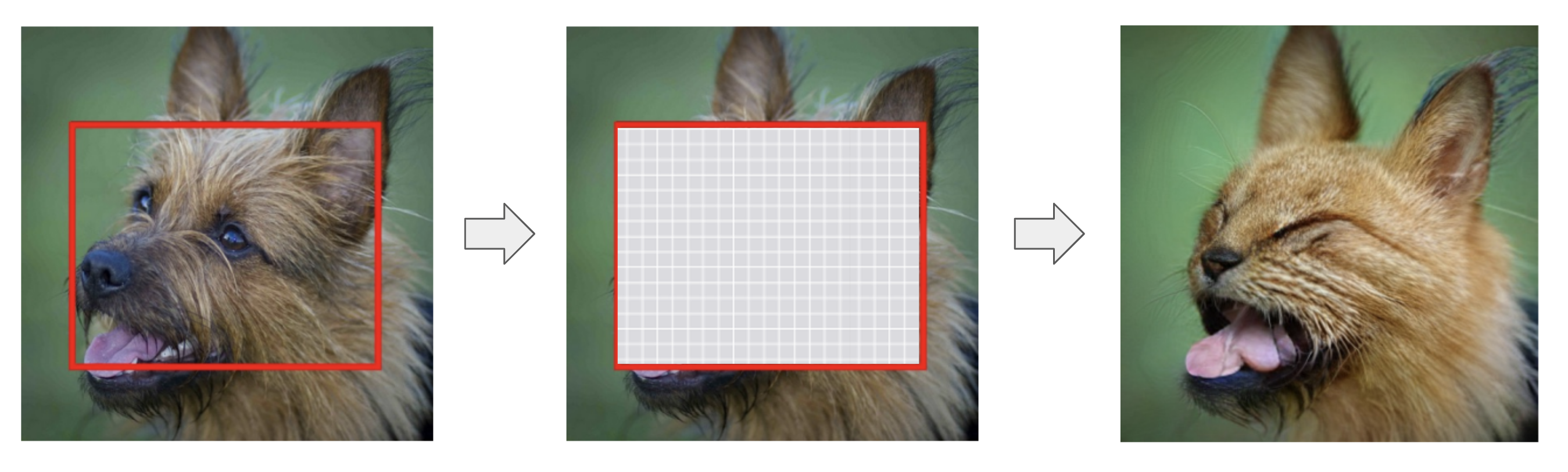Given an input image, target label and a bounding box automatically produced by the previous MaskRCNN Pipeline, we use a pretrained MaskGIT model for class-conditioned image editing. The region inside of the bounding box is considered as the initial mask to the MaskGIT iterative decoding algorithm.

The iterative decoding algorithm runs T steps. It starts with the initial mask, in our case, it's the rectangle gray area inside of the bounding box. Let the masked tokens be $Y_M^{(t)}$. At each step t, the model produces the image via predicting all tokens at the same time but only preserving the most confident ones. We define each masked location as $i$. A token $y_i^{(t)}$ is sampled based on its prediction probabilities $p_i^{(t)} \in \mathbf{R}^K$. The confidence score is defined as the corresponding prediction score of a token $y_i^{(t)}$, and it indicates how confident our model is of this prediction. For the non-masked regions, the confidence score is set to 1.0. For the less confident tokens, they are considered as the mask input to the next iteration, the mask ratio is designed to be decreasing so that within T iterations, all tokens are being generated.

After we perform this decoding algorithm for T iterations, the model generates a resulting image that has contents of the given target label and contexts of the input image.

In our example above, we see that given our original input image of a dog, target label cat and a bounding box, the contents within a box becomes the initial mask, and we obtain a cat image with the contexts (e.g. fur and color) that's similar to the original dog image.

### Fine-tuning with Text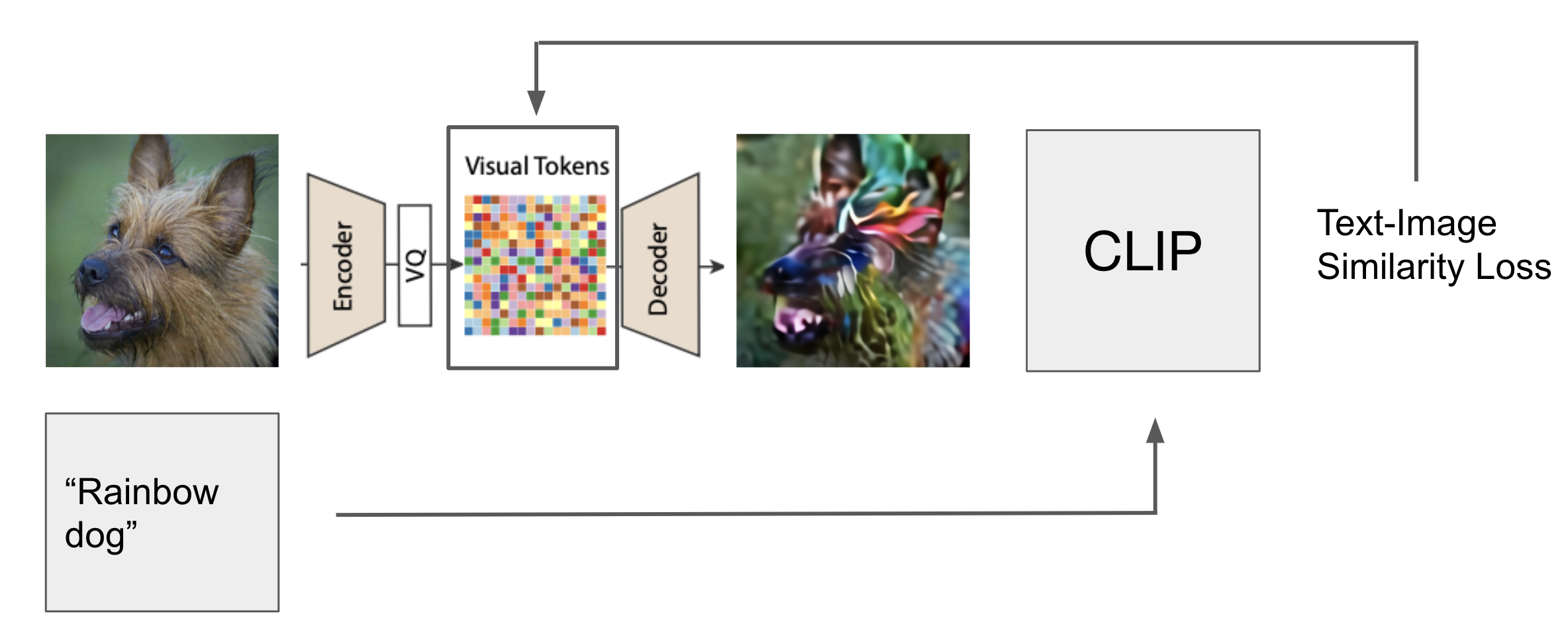Given a sample of class-conditioned image $x$, let $x = f_d(q_z)$ where $f_d$ is the VQ-VAE decoder and $q_z$ is the grid of quantized latent vectors. We optimize the text image similarity loss using pretrained CLIP model, CLIP($f_d(q_z)$, text_prompt). By fixing the decoder $f_d$, we optimize the text image similarity loss by performing gradient descent steps in $q_z$ space. This allows us to fine-tune the generated image on an open-domain text prompt. We see that pretrained CLIP model provides especially good guidance when we want to modify the color or texture of the generated images. However, if if we want to generate a new object from scratch, performing gradient descent on $q_z$ w.r.t. to CLIP loss does not lead to good results. In practice, we rely on pretrained MaskGIT to do content and object level image editing and we use CLIP optimization to control the texture of the output. When combined, MaskGIT + CLIP can produce a lot of creative edits in a huge space that is effectively 1,000 ImageNet classes * millions of potential texture and style adjective text prompts.

# Ablation

### The Effect of Bounding Box Size

While generating edited images with OpenGIT, we find that the output is particularly sensitive to the size of the bounding box, even if the bounding box always bounds the same object. Therefore, we introduce another parameter, $r$, the ratio of the size of the desired bounding box to that of the original bounding box given by MaskRCNN as described above. While rescaling the bounding box, we make sure both the center and the aspect ratio of the original bounding box are preserved. Here are some results produced by OpenGIT when we specify different $r$ while keeping other inputs the same.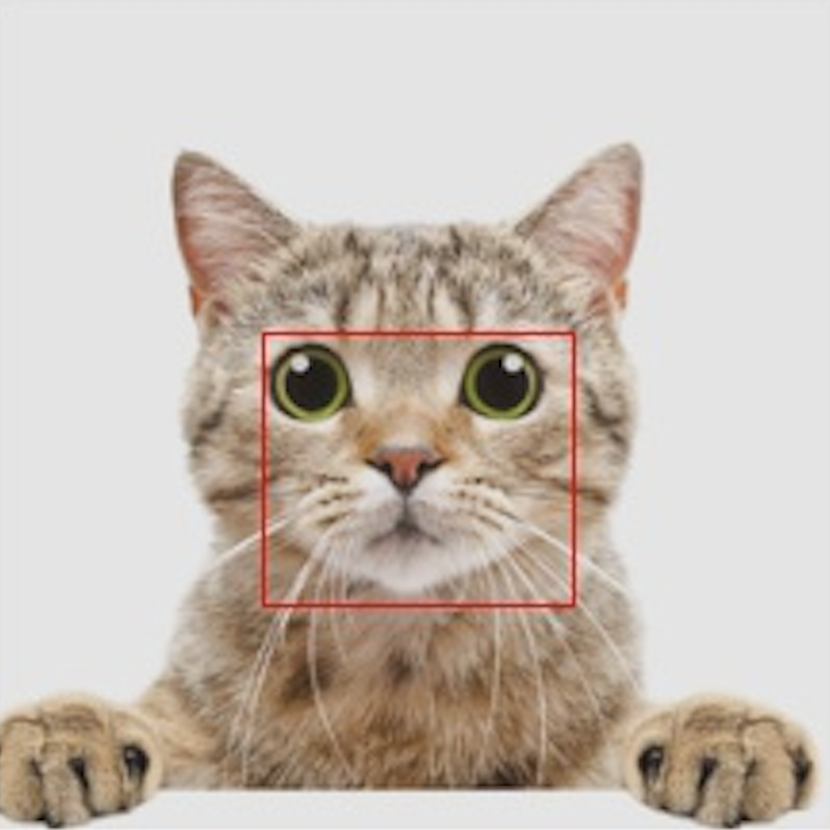Bounding box $r = 0.4$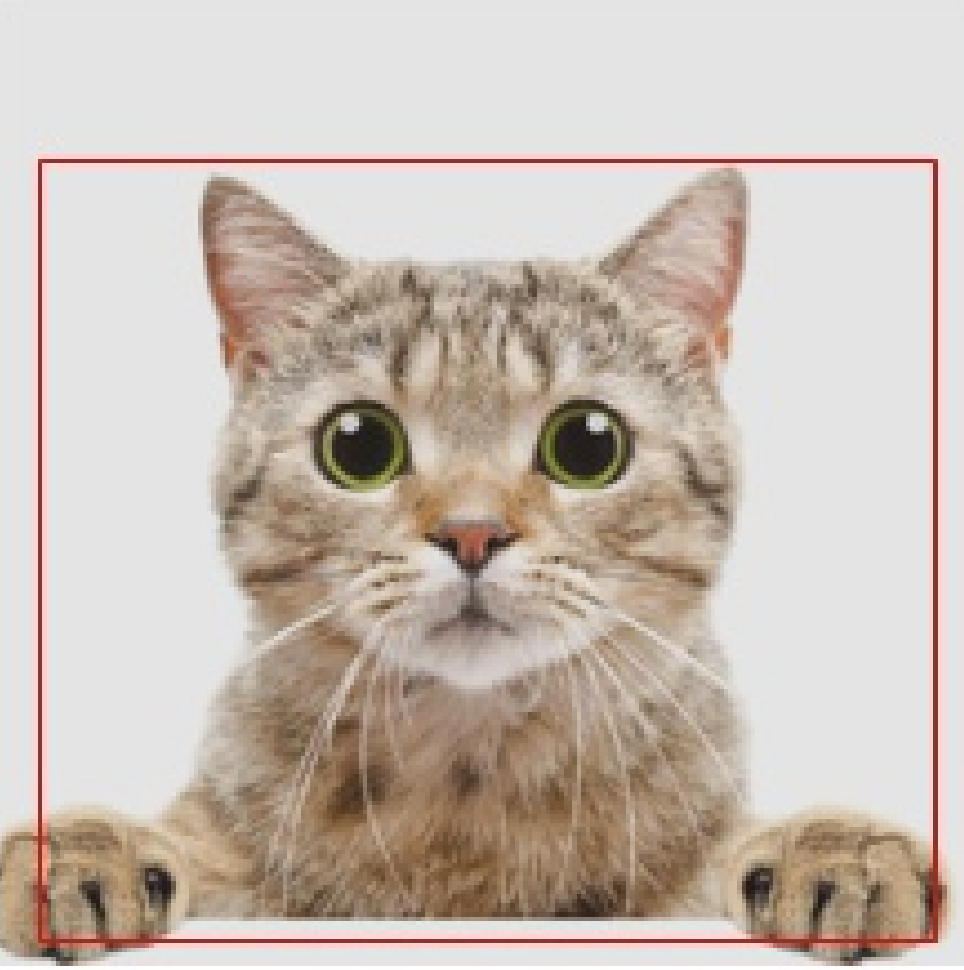Bounding box $r = 1$

To generate the above visualizations, we give the same prompt "change cat to rabbit". For the first example, when $r = 0.4$, the bounding box only covers the center part of the cat's face. It's expected that the model would produce a rabbit face that merges well with the original image. When $r = 1$, the whole cat is covered in the bounding box. In this case, the model replaces the cat with a rabbit, including ears and the body. Since there is no inherent quality difference between the two ways of editing and it should be up to the user to decide which output gives the desired image editing effect, we let users specify $r$ directly in our interface. Future work includes inferring $r$ automatically given descriptive words like "face only", "partially", "completely", etc.

# Results

Here're the results of our OpenGIT pipeline. Recall that the user inputs an image of an object with a prompt similar to "Replace {a} with {b}".

Firstly, we present the image generation for the case where {b} is simply a target category, thus no CLIP optimization is performed.
Next, we present the image generation for the case where {b} is a descriptive phrase about a target category, we perform CLIP optimization in this case.
Lastly, we present the results of {b} where b is a combination of different categories. Essentially, we achieve the results via combining the latent features of different categories.